# Please share some good analysis on Symmetrical circuits

@Shwetanshu_2018 bro please share some good analysis in symmatric circuits like
when we can use these,what should about resistance(perpendicular or bisector along symmatry line) what can be confusion what can be some trick and whatever you knows share every thing..
tell about delta star method same above point
thanks a lot..

@Viram_2019 sir, @pratyaksh_2019 sir please share ur concept too..

1 Like

There are primarily 2 types of symmetry

1. for eg you need to find equivalent across terminal A and B then place your hand along AB , if the circuit is symmetrical about AB then you can fold the circuit about line AB and suppose C’ was mirror image of C about AB then bow in this folded circuit they will be in parallel combination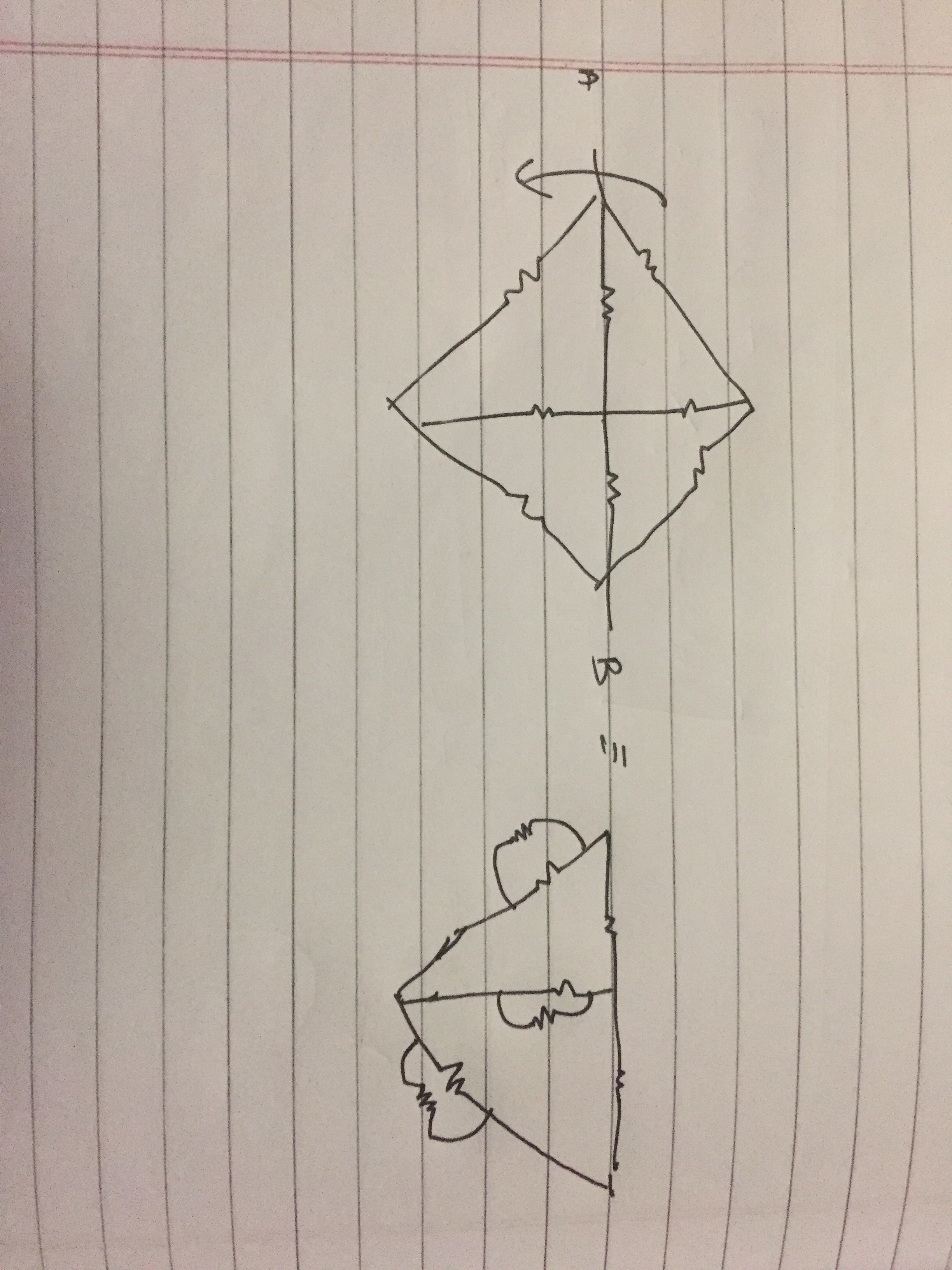1. when circuit is symmetrical about axis perpendicular to AB then place your hand along that perpendicular line and remove all those resistors which touches your hand and is perpendicular to it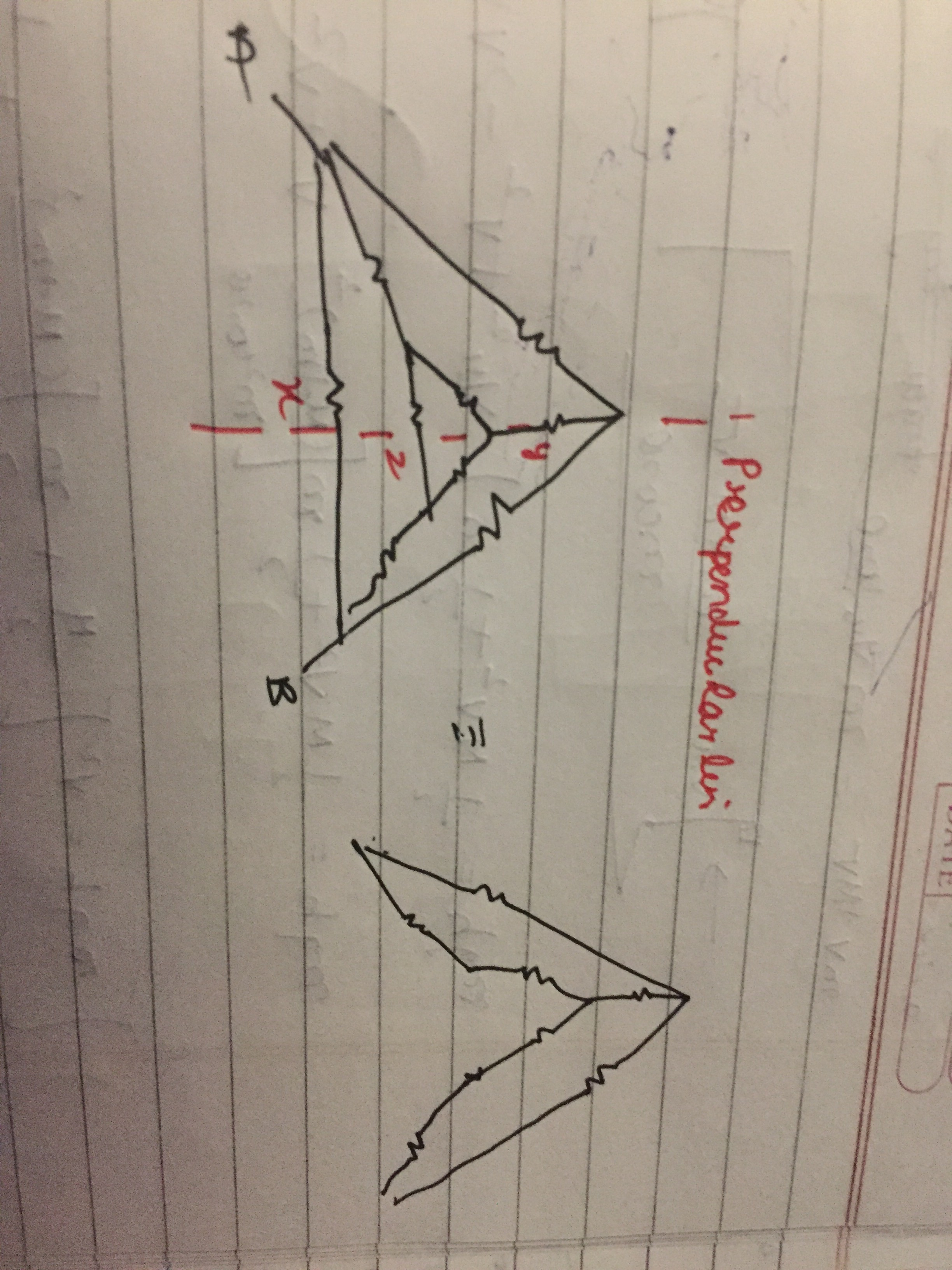Since x and z where perpendicular to perpendicular line , we can simply remove them , but y was along the perpendicular line so don’t remove it

For symmetrical there are basically only these 2
But sometime few other methods also helps us to simplify the circuit
For eg :- nodal method ( in this we write the potential of each point and then re- draw the circuit )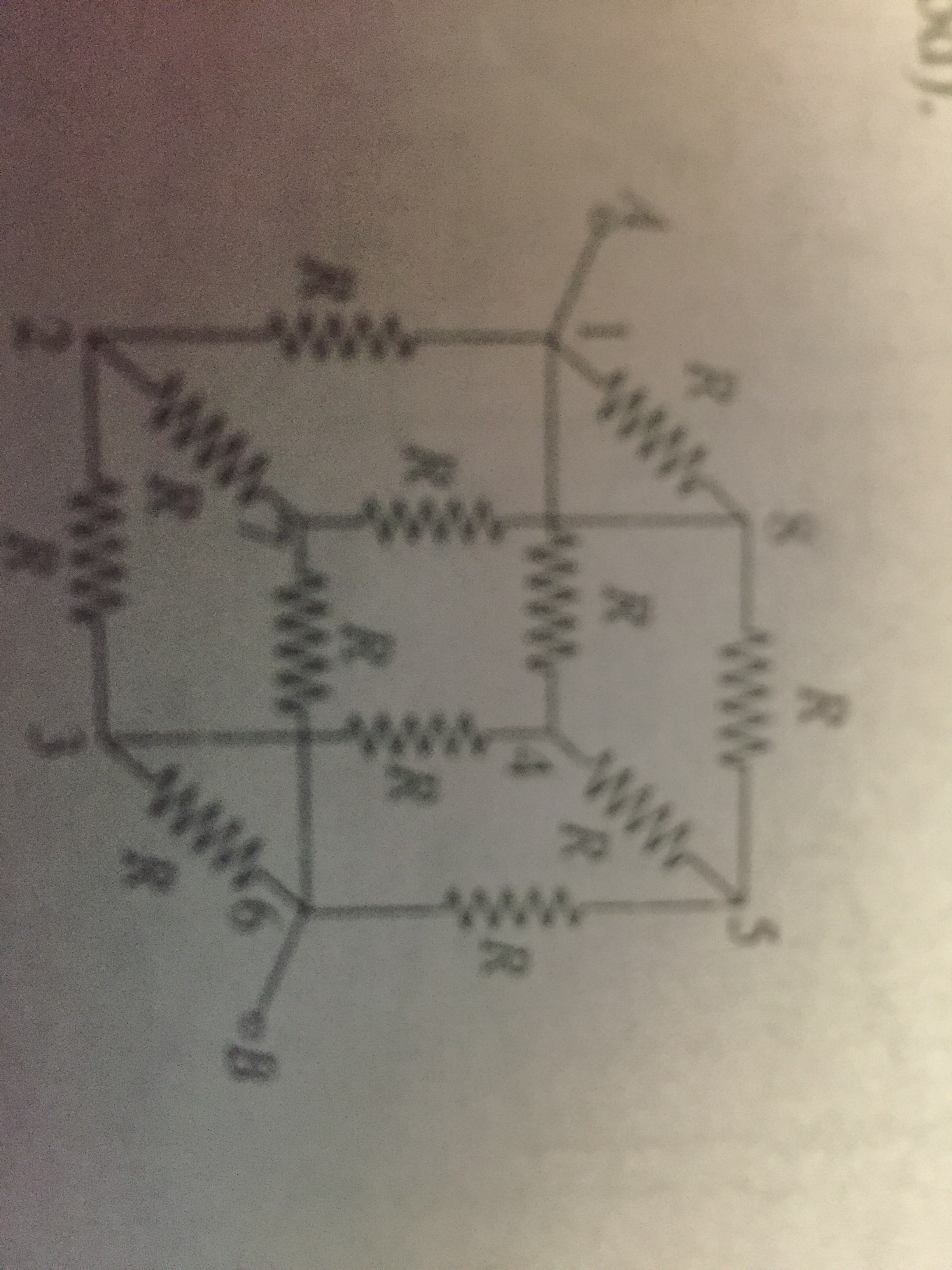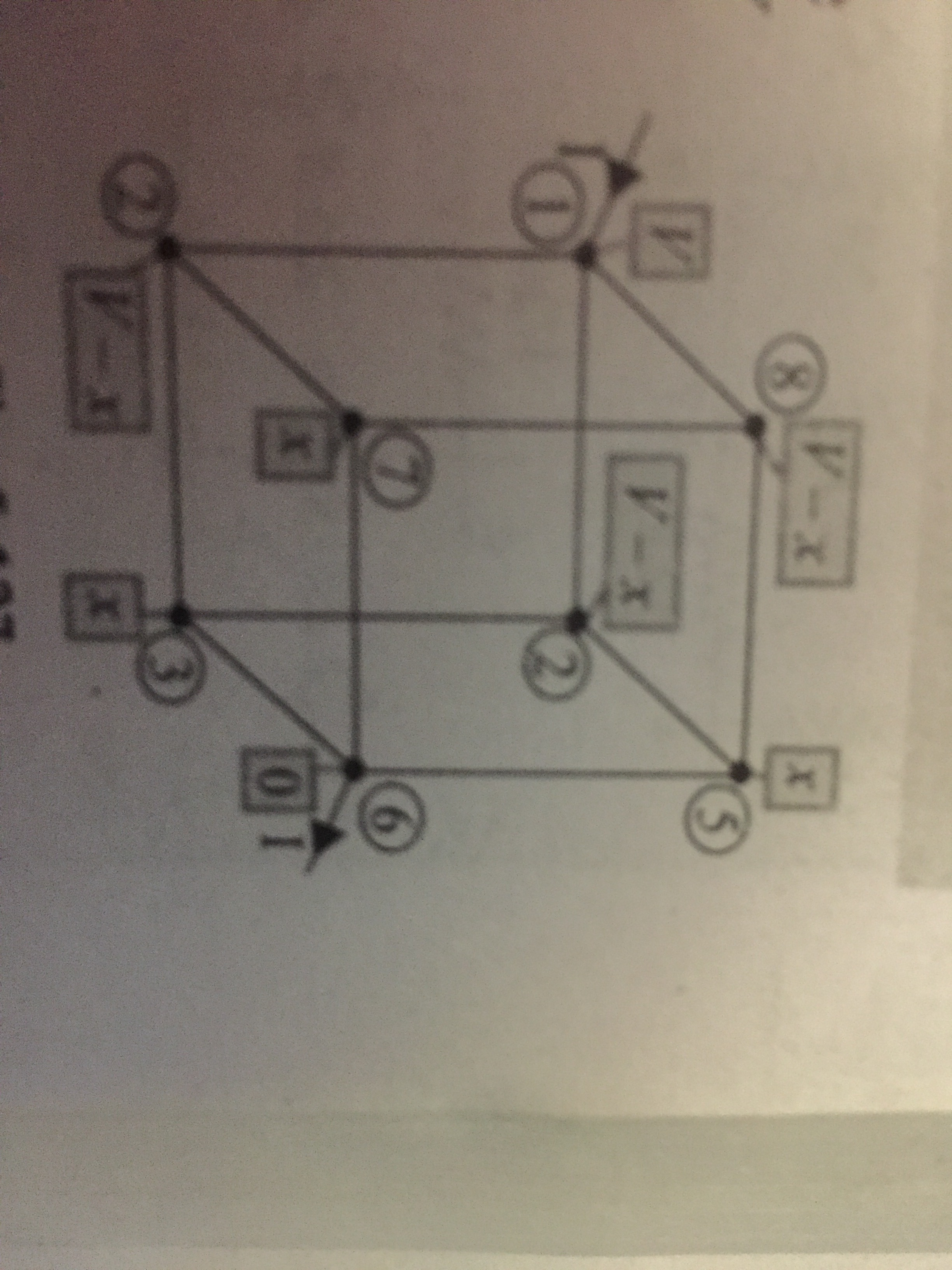In such problems , using symmetry , we write the potential of each point and then re draw a simplified circuit by joining the equipotential points

Another type of symmetry based question is when for example we have to find equivalent across A and B and there are points C and D in the circuit such that they have equivalent = equivalent across A and B or are multiple of it

Eg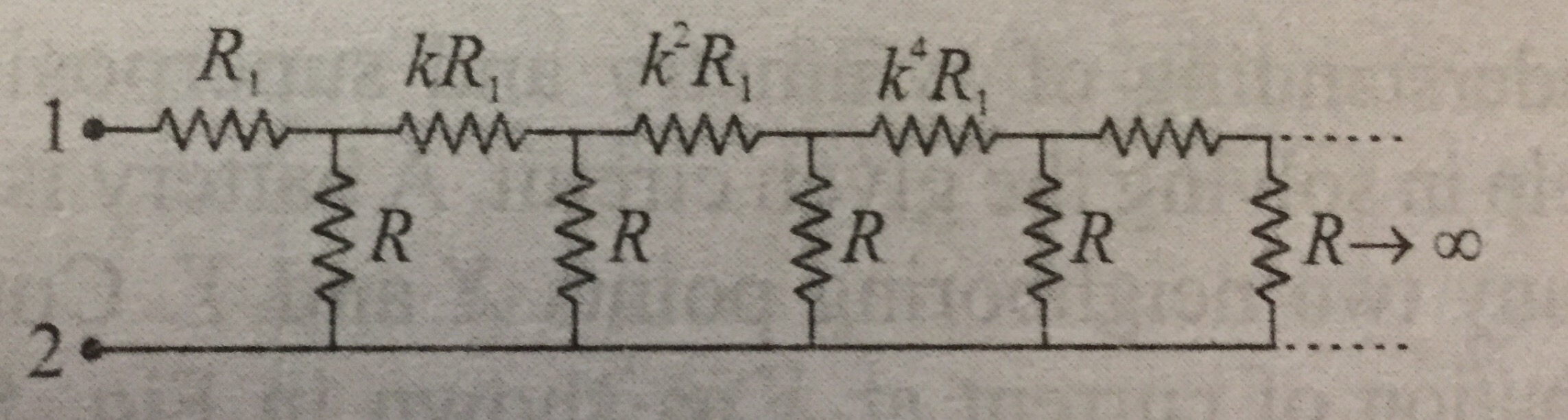Can be simplified as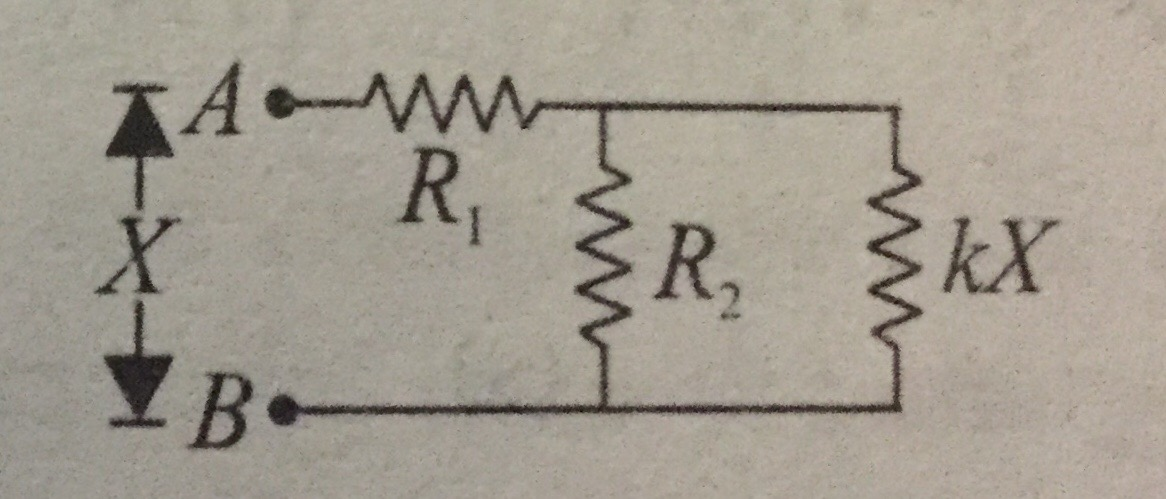Now you’ll get a quadratic in X and then you can get value of X

Next type of symmetry can be by distribution of current , this is nothing but an alternative method to visualise nodal method
For eg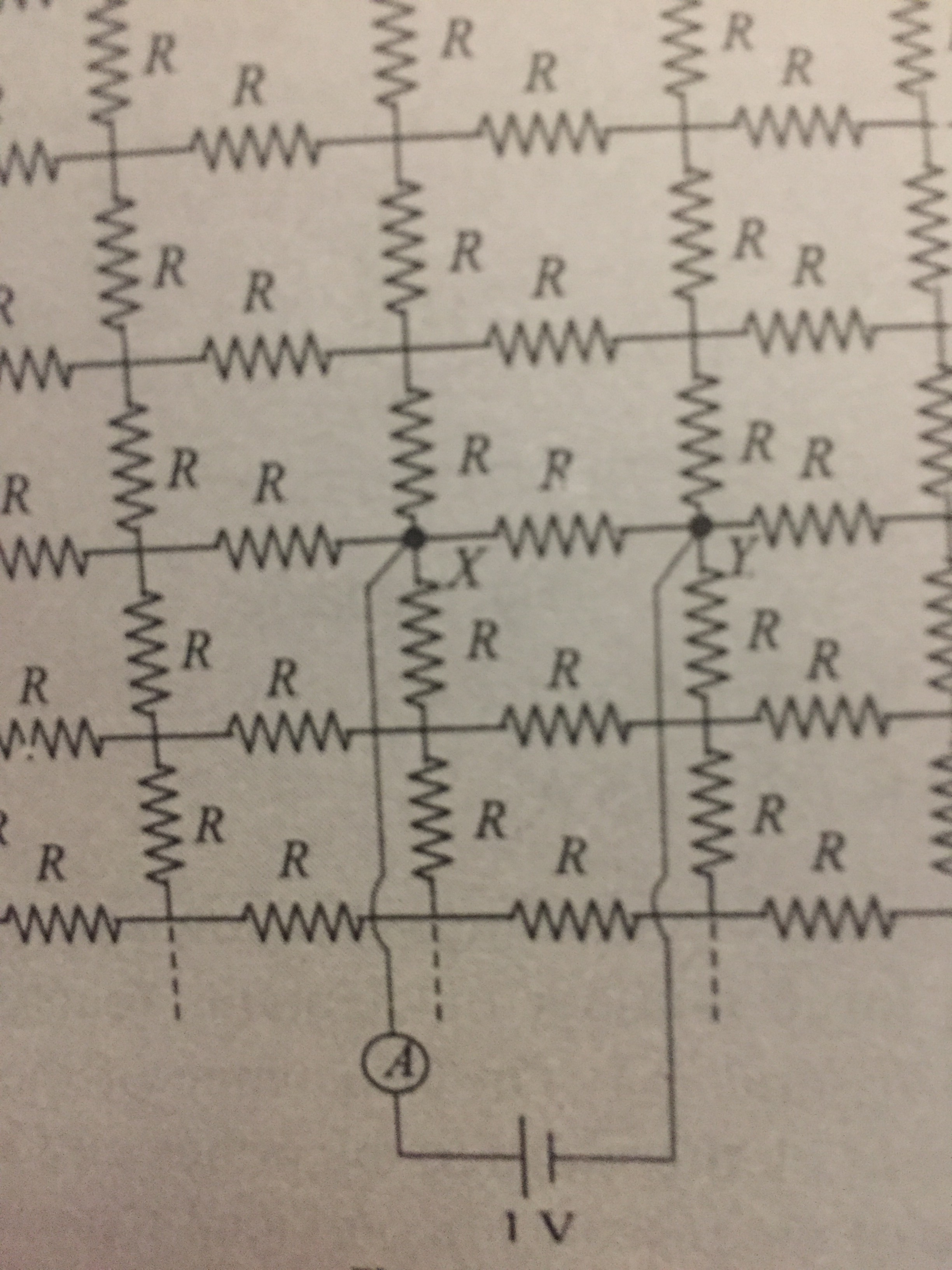In such questions you can see the current distribution and get the equivalent

Mostly all questions are based on these 5 (basically 4 as current method is an application of nodal method) or combination of these .

Star to Delta and delta to star conversion is not there in JEE syllabus , so you’ll never require the knowledge of it for questions if JEE but still if you need to know about it , then do let me know , I’ll write a post on how to convert star to Delta and vice-versa

yes sir i want to know for my learning sir if time you may share sir

Delta to star conversion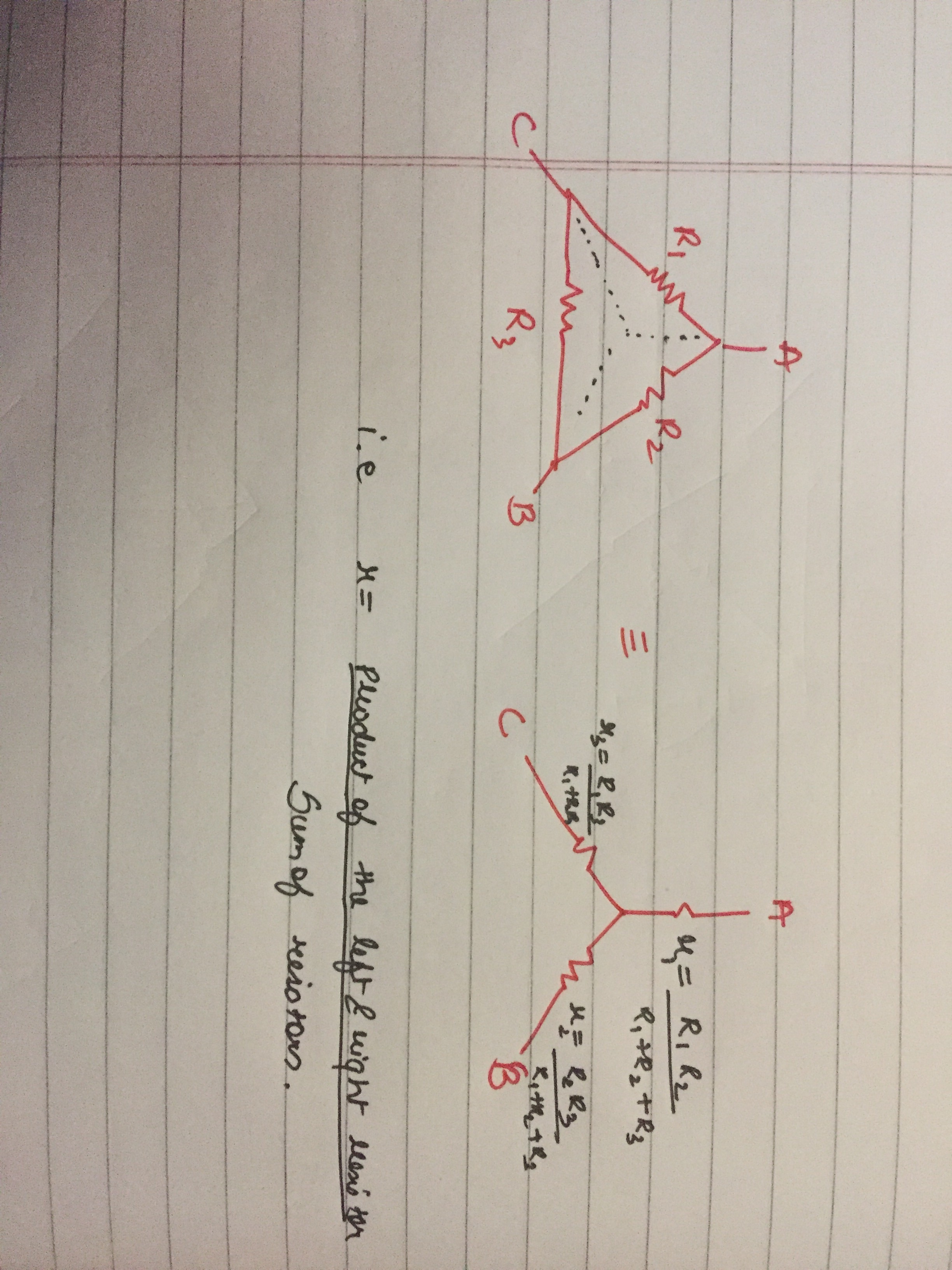1 Like

Star to delta conversion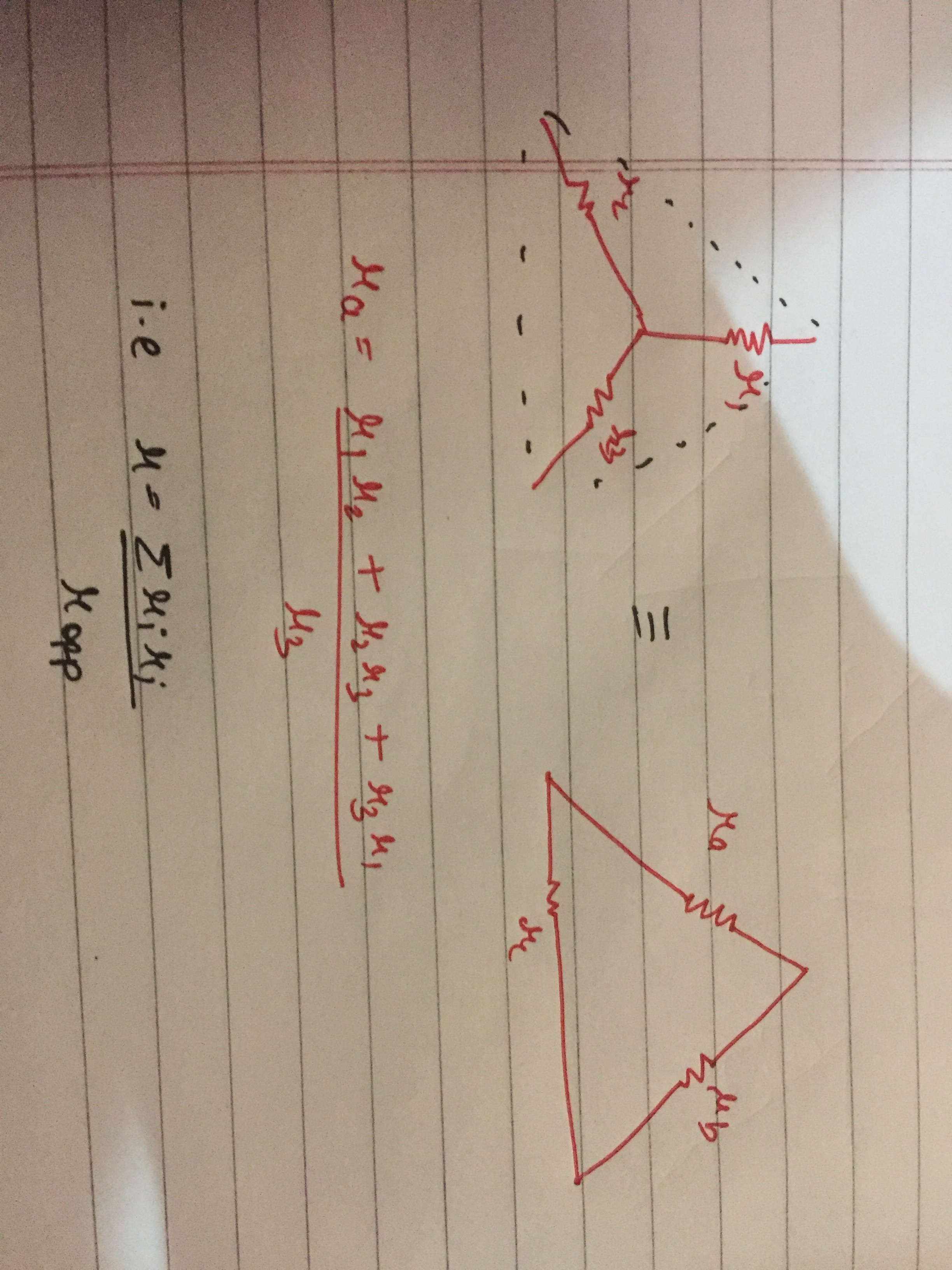These should suffices for most of the questions i think !

1 Like